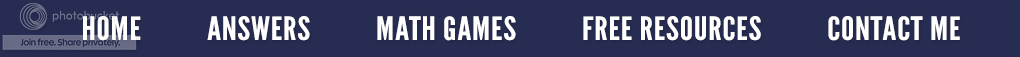### The Mysterious Case of Zero, the Exponent - Why any Number to the Zero Power Equals One

Sometimes my college students like to ask me what seems to be a difficult question. (In reality, they want to play Stump the Teacher.)  I decided to find out what sort of answers other mathematicians give; so, I went to the Internet and typed in the infamous question, "Why is any number to the zero power one?"  It was no surprise to find numerous mathematically correct answers, most written in what I call "Mathteese" - the language of intelligent, often gifted math people, who have no idea how to explain their thinking to others.  I thought, "Wow!  Why is math always presented in such complicated ways?"  I don't have a response to that, but I do know how I introduce this topic to my students.

Since all math, and I mean all math, is based on patterns and not opinions or random findings, let's start with the pattern you see on the right.  Notice in this sequence, the base number is always 3.  The exponent is the small number to the right and written above the base number, and it shows how many times the base number, in this case 3, is to be multiplied by itself.

(Side note: Sometimes I refer to the exponent as the one giving the marching orders similar to a military commander. It tells the base number how many times it must multiply itself by itself. For those students who still seem to be in a math fog and are in danger of making the grave error of multiplying the base number by the exponent, have them write down the base number as many times as the exponent says, and insert the multiplication sign (×) between the numbers. Since this is pretty straight forward, it usually works!)

Notice our sequence starts with 31 which means 3 used one time; so, this equals three; 32 means 3 × 3 = 9, 33 = 3 × 3 × 3 = 27, and so forth. As we move down the column, notice the base number of 3 remains constant, but the exponent increases by one. Therefore, we are multiplying the base number of three by three one additional time.

Now let's reverse this pattern and move up the column. How do we get from 243 to 81? That's right! We divide by three because division is the inverse operation (the opposite) of multiplication. How do we get from 243 to 81? That's right! We divide by three because division is the inverse operation (the opposite) of multiplication. Notice as we divide each time, the exponent by the base number of 3 is reduced by one. Let's continue to divide by three as we move up the column. 27÷ 3 = 9; 9 ÷ 3 = 3. Now we are at 31 = 3 which means we must divide 3 by 3 which gives us the quotient of 1. Notice, to fit our pattern, the 3 in the left hand column would have to be 30 ; so, 30 must equal one!

This works for any number you wish to put in the left hand column. Try substituting the base number of three with two. Work your way up the sequence dividing by two each time. You will discover that two divided by two equals one (20 = 1). Therefore, we can conclude that any natural number with an exponent of 0 is equal to one. (Zero is not included; it's another mystery to solve.)What happens if we continue to divide up the column past 30 ?   (Refer back to the sequence on the left hand side.)  Based on the pattern, the exponent of zero will be one less than 0 which gives us the base number of 3 with a negative exponent of one or 3-1 .    To maintain the pattern on the right hand side, we must divide 1 by 3 which looks like what you see on the left. Continuing up the column and keeping with our pattern, 3 must now have a negative exponent of 2 or 3-2 and we must divide 1/3 by 3 which looks like what is written on the right.

Each time, the exponent by the base number of 3 is reduced by one. Let's continue to divide by three as we move up the column. 27÷ 3 = 9; 9 ÷ 3 = 3. Now we are at 31 = 3 which means we must divide 3 by 3 which gives us the quotient of 1. Notice, to fit our pattern, the 3 in the left hand column would have to be 30 ; so, 30 must equal one! This works for any number you wish to put in the left hand column. Try substituting the base number of three with two. Work your way up the sequence dividing by two each time. You will discover that two divided by two equals one (20 = 1). Therefore, we can conclude that any natural number with an exponent of 0 is equal to one. (Zero is not included; it's another mystery to solve.)

As a result, the next two numbers in our pattern are..............

Isn't it amazing how a pattern not only answers the question: "Why is any number to the zero power one?" But it also demonstrates why a negative exponent gives you a fraction as the answer. (By the way math detectives, do you see a pattern with the denominators?)

Mystery Solved!   Case Closed!

This lesson is available on a video entitled:  Why Does "X" to the Power of 0 Equal 1?Only \$3.75
----------------------------------------------------------------------------------------

Want simple, visual answers to other difficult math questions? Try this resource entitled Six Difficult Math Questions with Answers. Many of the answers feature a supplementary video for a more detailed explanation.